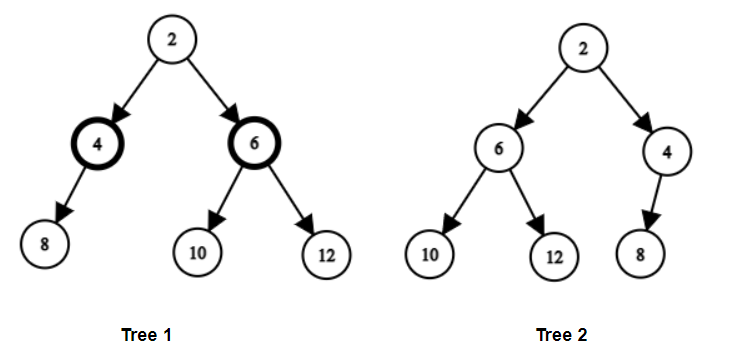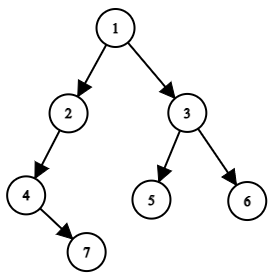# Flip Equivalent Binary Tree

Posted: 12 Mar, 2021
Difficulty: Moderate

## PROBLEM STATEMENT

#### A flip operation is defined as: Choose any node and swap the left and right subtrees of that node.

##### Note:
``````All the values of the tree are unique.
``````
##### For Example:
``````For the given binary trees
````````````Tree 1 can be made flip equivalent to Tree 2 by flipping the left and right sub trees of the node with value = 2.
``````
##### Input Format:
``````The first line contains an integer 'T' which denotes the number of test cases. Then the test cases are as follows.

The first and second line of each test case contains elements of the tree in the level order form. The line consists of values of nodes separated by a single space. In case a node is null, we take -1 in its place.
````````````For example, the input for the tree depicted in the above image would be :

1
2 3
4 -1 5 6
-1 7 -1 -1 -1 -1
-1 -1
``````

#### Explanation :

``````Level 1 :
The root node of the tree is 1

Level 2 :
Left child of 1 = 2
Right child of 1 = 3

Level 3 :
Left child of 2 = 4
Right child of 2 = null (-1)
Left child of 3 = 5
Right child of 3 = 6

Level 4 :
Left child of 4 = null (-1)
Right child of 4 = 7
Left child of 5 = null (-1)
Right child of 5 = null (-1)
Left child of 6 = null (-1)
Right child of 6 = null (-1)

Level 5 :
Left child of 7 = null (-1)
Right child of 7 = null (-1)
``````
##### Note:
``````The above format was just to provide clarity on how the input is formed for a given tree.
The sequence will be put together in a single line separated by a single space. Hence, for the above-depicted tree, the input will be given as:

1 2 3 4 -1 5 6 -1 7 -1 -1 -1 -1 -1 -1
``````
##### Output Format:
``````For each test, print “Yes” if the two trees are flip equivalent. Otherwise, print “No”.

Print the output of each test case in a separate line.
``````
##### Note:
``````You do not need to print anything, it has already been taken care of. Just implement the given function.
``````
##### Constraints:
``````1 <= T <= 50
0 <= N <= 10^3
1 <= DATA <= 10^4

Where ‘N’ is the total number of nodes in the binary tree, and “DATA” is the value of the binary tree node.

Time Limit: 1 sec
``````Approach 1

Approach: A simple idea to check if the tree is flip equivalent or not is to use recursion. We will recursively check for every node.

1. Let flipEquivalent( ROOT1, ROOT2 ) be the recursive function.
2. If ROOT1 and ROOT2 both are null, then we will simply return true.
3. If either ROOT1 is null, ROOT2 is null or ROOT1 -> DATA is not equal to ROOT2-> DATA, return false as we can not make the trees flip equivalent in any of these cases.
4. If ROOT1 and ROOT2 have the same data, then we will check for their child nodes. There will be two cases for that.
• Either we need to flip the left and right sub-tree of root1 or there will be no need for that.
• If we flip left and right subtree we will recursively check
• flipEquivalent( ROOT1 -> LEFT, ROOT2 -> RIGHT ) && flipEquivalent( ROOT1→ RIGHT, ROOT2 -> LEFT )
• If we do not flip, we will check  flipEquivalent( ROOT1 -> LEFT,  ROOT2 -> LEFT ) && flipEquivalent( ROOT1 -> RIGHT, ROOT2 -> RIGHT ).
• Return bitwise OR  of the above two cases.

Algorithm:

1. If ‘ROOT1’ = NULL and ‘ROOT2’ = NULL, return “true”.
2. If ‘ROOT1’ = NULL or ‘ROOT2’ = NULL or ‘ROOT1 → DATA' ! = ‘ROOT2 → DATA’, return "false".
3. Return ( flipEquivalent( ROOT1 -> LEFT, ROOT2 -> RIGHT ) &&  flipEquivalent( ROOT1 -> RIGHT, ROOT2 -> LEFT)  Or  flipEquivalent( ROOT1 -> LEFT, ROOT2 -> LEFT ) &&  flipEquivalent( ROOT1 -> RIGHT, ROOT2 -> RIGHT ).